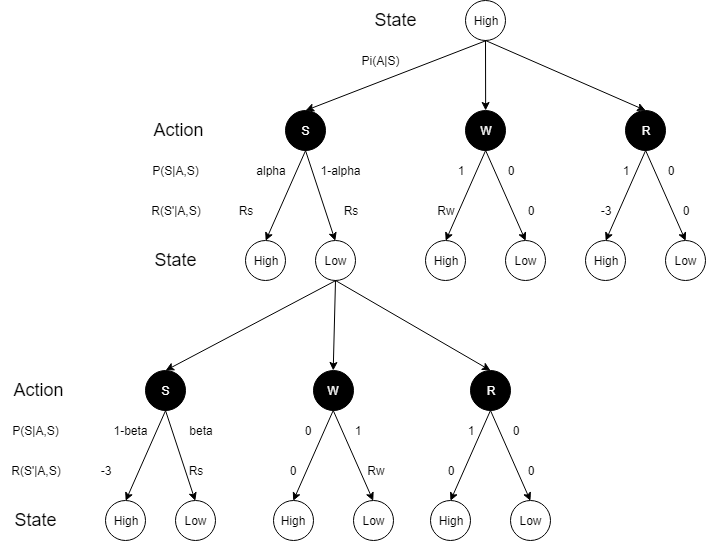# 【RL系列】马尔可夫决策过程——状态价值评价与动作价值评价

【RL系列】马尔可夫决策过程中状态价值函数的一般形式

【RL系列】马尔可夫决策过程与动态编程

GridWorld• 将该地图中的16个格子分别记为16种状态，建立奖励机制，给出各个状态的奖励：目的地状态奖励设为1，其它状态奖励设为0，其奖励矩阵可以写为$\mathbf{R}$： $$\mathbf{R} = \left[ \begin{matrix} 1 & 0 & 0 & 0 & 0 & 0 & 0 & 0 & 0 & 0 & 0 & 0 & 0 & 0 & 0 & 1 \end{matrix}\right]$$
• 使用状态价值评价函数求解每个状态收敛时的价值。
• 依据各个状态的价值制定policy，以greedy-policy为例，每次做出前往当前状态周边价值最大的状态的动作。

• 在迭代过程中，记Agent每做一个动作，奖励为-1
• 目的地的动作选择概率设为0，即在目的地则不作任何动作
• 使用bellman方程来收敛计算价值评价
• 通过动作价值或状态价值计算policy

可以发现两者的不同主要体现在奖励机制的不同，奖励机制的不同也影响了价值评价函数的不一样。bellman方程所使用的奖励机制为动作奖励机制，即给每个动作规定该动作可以获得的奖励，这是典型的AVE的思想。AVE和SVE的最大不同点在于，SVE是用当前状态所获得的奖励与未来可能到达的状态的价值（未来价值）去评价当前状态的价值，而AVE是用未来可能动作价值的期望去评价当前状态的价值，而未来动作价值的期望又是由未来可能奖励的期望与未来可能状态的价值期望的线性组合。

$$\mathbf{V}(s) = \mathbf{R}(s) + \gamma \mathbf{V}'$$

AVE的价值更新函数可以写为：

$$\mathbf{V} = \sum_{a} \pi(a) \mathbf{Q}(a)$$

$$\mathbf{Q}(a) = \mathbb{R}(a) + \gamma \mathbf{V}'$$

Recycling Robot

“回收机器人”是一个典型的动作价值评价问题，如需详细了解这个问题，可以阅读Reinforcement Learning: An Introduction(2017)中的第三章。执行主体为回收机器人，其状态可分为两种，高电量状态(Hight)和低电量状态(Low)。可选择的动作有三种，搜索(Search)，等待(Wait)和充电(Recharge)。不同的动作也会依一定的概率分布产生新的状态。为了更直观的理解这个问题，这里我们可以画出Recycling Robot的状态转移图像：由于在这个问题里，动作与状态不仅不能够实现一一对应，而且该决策过程并没有一个或多个的目标状态，是无法使用SVE方法来解决这个问题的，但bellman方程确是合适的。“回收机器人”的目的并非达到某个目的状态，而是做出符合获取最大利益的决策（动作），所以下面我将给出具体的状态转移概率矩阵与动作奖励矩阵，尝试解决这个决策问题。

• 设$\alpha = 0.8$，也就是说在High状态下做出Search动作可保持High状态的概率为0.8，所以电量降低到Low状态的概率为0.2，此时的奖励两者皆为$R_s = 1$
• 设$\beta = 0.15$，说明在Low状态下做出Search动作可保持Low状态的概率为0.15，获得的奖励为$R_s = 1$。没电宕机的概率为0.85，此时需要人为救助重新回到High状态，奖励为$R_s = -3$
• 设在两种装填下选择Wait动作可获得的收益为$R_w = 0.5$，注意在做Wait动作后，当前状态不会改变，可以保持。
• Recharge动作可以使得当前状态转变为High状态。在High状态下做Recharge动作获得的奖励为$R_r = -3$，在Low状态下做Recharge动作不获得任何奖励，记$R_r = 0$

\begin{align} & R(High) & = \left[\begin{matrix} 1 & 0.2 & -3\\ 1 & 0 & 0 \end{matrix}\right]\\ & R(Low) & = \left[\begin{matrix} -3 & 0 & 0\\ 1 & 0.2 & 0 \end{matrix}\right]\\ &P(High) &= \left[\begin{matrix} 0.8 & 0.2 \\ 1 & 0 \\ 1 & 0 \end{matrix}\right]\\ &P(Low) &= \left[\begin{matrix} 0.15 & 0.85 \\ 0 & 1 \\ 1 & 0 \end{matrix}\right] \end{align}

$$\mathbf{R} = \mathrm{fold}\left[\begin{matrix} R(High)\\R(Low) \end{matrix}\right]\\ \mathbf{P} = \mathrm{fold}\left[\begin{matrix} P(High)\\P(Low) \end{matrix}\right]$$

$$\mathbb{R} = \mathbb{E}(A|S = Low) = \mathrm{diag}\left(\left[\begin{matrix} 0.15 & 0.85 \\ 0 & 1 \\ 1 & 0 \end{matrix}\right] \left[\begin{matrix} -3 & 0 & 0\\ 1 & 0.2 & 0 \end{matrix}\right] \right) = \left[ \begin{matrix} -2.4\\ 0.2\\ 0\end{matrix}\right]$$

\begin{align} &\mathbf{V} = \mathbf{\Pi} \mathbf{Q} \\ &\mathbf{Q} = \mathrm{diag}(\mathbf{P}\mathbf{R}) + \gamma \mathbf{P}\mathbf{V}' \end{align}

\begin{align} & \mathbf{V} = \mathbf{R}_{s} + \gamma \mathbf{\Pi}\mathbf{Q} \\ & \mathbf{Q} = \mathrm{diag}(\mathbf{P} \mathbf{R}_{s', a}^{s}) + \gamma \mathbf{P} \mathbf{V}' \end{align}

$$\pi = \begin{matrix} H\\L \end{matrix} \left[\begin{matrix} P(\mathrm{Search}) & P(\mathrm{Wait}) & P(\mathrm{Recharge}) \\ P(\mathrm{Search}) & P(\mathrm{Wait}) & P(\mathrm{Recharge}) \end{matrix}\right] =\left[\begin{matrix} 0.8198 & 0.1799 & 0.0003\\0.0039 & 0.4937 & 0.5024\end{matrix}\right]$$

$$\pi = \left[\begin{matrix} 0.7879 & 0.2118 & 0.0004\\ 0.0049 & 0.2614 & 0.7337 \end{matrix}\right]$$

$$\pi = \left[\begin{matrix} 0.8514 & 0.1483 & 0.0002\\0.0022 & 0.7552 & 0.2426 \end{matrix}\right]$$

posted @ 2018-07-13 20:24 JinyuBlog 阅读(...) 评论(...) 编辑 收藏